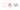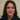# How to generate a mappable array in Javascript

Doing researches around new Javascript techniques I came across this technique from Shawn Reisner of how to generate a mappable array in Javascript. Let me explain it.

## Generate an array

Basically we create arrays in the old way like the code below. If we want to create an array of numbers for example:

``````const arr = [];
for (let i = 0; i < 100; i++) {
arr[i] = i;
}``````

Generally this is correct and works as expected. We will get an array of 100 numbers starting from 0 to 99. But as we learnt about higher order functions and map and other ES6 features we also want to do this in ES6 way.

## Create a mappable array

Luckily we have higher order functions to help us and the best option is map method. We can just create a mappable array of length 100 like this:

``const arr = Array(100);``

And map each index to a number using map:

``const arr = Array(100).map((_, i) => i);``

As a result this is what we get:Surprisingly we get undefined. As an explanation we know arrays are objects in practice. So the array like  [‘one’, ‘two’, ‘three’] is equivalent to this object:

``````{
0: 'one',
1: 'two',
2: 'three',
length: 3
}``````

Each array item has a key and value. When we create a new array with the Array constructor, it creates a new array object with its length _property set to the value you passed in, but otherwise _the object is a vacuum.

So in our case we actually have an array with no keys with undefined  values and only the length of 100:

``````{
//no index keys!
length: 100
}``````

Therefor to make a mappable array we need to have keys even if the value is empty of in terms of Javascript “undefined”. In map the callback is only executed _if the key exists _on the object.  The default behaviour in JavaScript is to return _undefined _if you try to access the value of an object for a key that does not exist.

## A truly mappable array

The best way to do this is to spread the array out into an empty array. Then look at the result:

``````const arr = [...Array(100)].map((_, i) => i);
console.log(arr);
// 0``````

Spread operator actually creates a copy with indexes for all array items. It simply loops through the array from 0 to _length _and creates a new index key in the enclosing array with the value returned from the spreading array at the current index.:

``````{
0: undefined,
1: undefined,
2: undefined,
...
99: undefined,
length: 100
}``````

Therefor we will have an array with key value pairs although the values are undefined as a Javascript default for empty arrays. But this time we have a mappable array that we can easily manipulate. Thanks for reading.